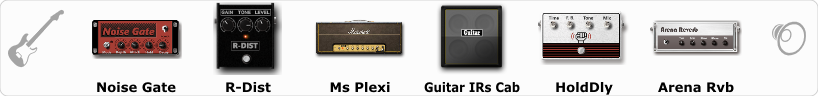Discussion in 'ToneLib-GFX presets' started by Pep, Jul 20, 2019.

Preset name: Heavy Metal Plexi Lead

Effects chain:Effect: "Noise Gate" (Dynamics / Filter), active - "yes"
"Detect" = Gtr In
"Mode" = Manual
"Depth" = 20
"Threshold" = 86
"Attack" = 0
"Hold" = 5
"Decay" = 250

Effect: "R-Dist" (Overdrive / Distortion), active - "yes"
"Drive" = 33
"Tone" = 80
"Level" = 66

Effect: "Ms Plexi" (Amp simulators), active - "yes"
"Gain" = 60
"Bass" = 100
"Middle" = 100
"Treble" = 100
"Presence" = 50
"Master" = 100
"Output" = 50
"Level (dB)" = 0

Effect: "Guitar IRs Cab" (Cabinets), active - "yes"
"Model" = Marshall 1960A (4x12")
"Mic Position" = Middle
"Mic Distance" = Middle
"Low Cut (Hz)" = 60
"Hi Cut (kHz)" = 20.0
"Mix" = 100
"Level (dB)" = 2

Effect: "HoldDly" (Delay), active - "yes"
"Time" = Note sync, 1/8 Dotted
"Feedback" = 45
"Tone" = 100
"Mix" = 35
"Hold" = Off

Effect: "Arena Rvb" (Reverb), active - "yes"
"Time" = 4.0
"PreDelay" = 23
"LoDamp" = 0
"HiDamp" = 0
"Mix" = 25

Note: You will need to download and install the ToneLib-GFX software to use the preset.